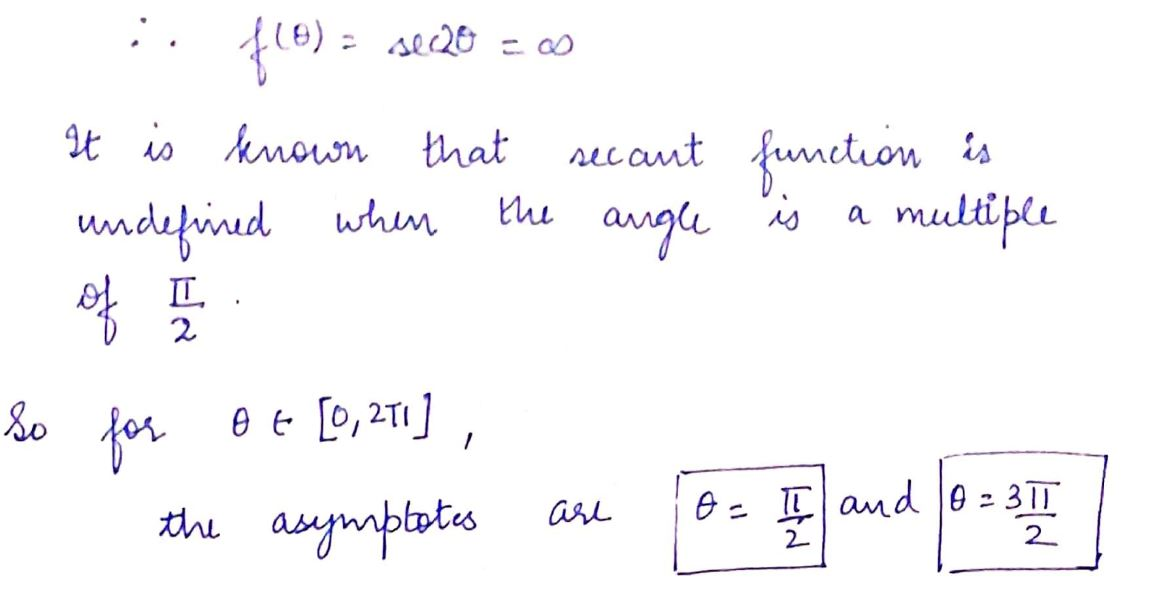# Consider f(θ) =sec(2θ)How many asymptotes can be found  over the domain of θ ∈ [0,2pi]? State their locations.Give an equation  that predicts  the location of any asymptote for f(θ) given that  θ ∈ R

Question
1 views

Consider f(θ) =sec(2θ)

How many asymptotes can be found  over the domain of θ ∈ [0,2pi]? State their locations.

Give an equation  that predicts  the location of any asymptote for f(θ) given that  θ ∈ R

check_circle

Step 1Step 2...

### Want to see the full answer?

See Solution

#### Want to see this answer and more?

Solutions are written by subject experts who are available 24/7. Questions are typically answered within 1 hour.*

See Solution
*Response times may vary by subject and question.
Tagged in